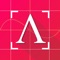You are using an outdated browser. Please upgrade your browser to improve your experience.## Take a math problem photo and Algebrator will do the rest# Algebrator: Camera Math Solver

by Softmath### What is it about?

Take a math problem photo and Algebrator will do the rest!### App Details

Version
1.4.8
Rating
(33)
Size
26Mb
Genre
Education Reference
Last updated
March 12, 2021
Release date
February 11, 2020
More info

### App Screenshots### App Store Description

Take a math problem photo and Algebrator will do the rest!
The Algebrator Camera Calculator has been helping high-school and college students conquer math for more than three decades. Learn how to solve math problems by seeing them worked out step-by-step.

Algebrator lets you either manually enter or scan any problem from your math or algebra textbook and gives you all steps to the solution, as well as explanations for each step. You’ll not only see the answer, you’ll understand how it got there.

This easy-to-use calculator app solves math problems just like your teacher would! The explanations will tell you not only which algebra rule is being used, but how it is applied in your particular math problem.

Whether you need help with math homework or want to better understand algebra, Algebrator will show you how it’s done. Here are some of the things Algebrator will do for you:

STEP-BY-STEP MATH CALCULATOR
- Show step-by-step solutions to any algebra problem you enter.
- Modify the details of the solution process you see, as well as the way in which a problem is solved.
- Gain an understanding of how each problem is solved.

ALGEBRA CALCULATOR
- Solve a variety of math equations and inequalities (linear, non-linear, quadratic, rational, radical and absolute value), as well as systems of equations or inequalities.
- Simplify any algebraic expression and factor polynomials using a variety of methods.
- Find the Greatest Common Factor (GCF) and the Least Common Measure (LCM) of multiple expressions.

SOLVE ARITHMETIC AND ALGEBRA PROBLEMS
- Integers and decimal numbers.
- Fractions.
- Roots.
- Powers.
- Exponents.

GRAPHING CALCULATOR APP
- Graph equations, inequalities, functions (including logarithmic, exponential and trigonometric) as well as conic sections (parabolas, circles, ellipses and hyperbolas).
- Perform common operations on matrices and calculate determinants.

If you need help with math, then Algebrator is the right way to go. Softmath has provided math and algebra help to high school and college students since 1990.

Download Algebrator today and see why students and teachers trust Softmath!

Terms of Use: https://softmath.com/termsofuse/
Privacy Policy: https://softmath.com/privacy/

Disclaimer:
AppAdvice does not own this application and only provides images and links contained in the iTunes Search API, to help our users find the best apps to download. If you are the developer of this app and would like your information removed, please send a request to [email protected] and your information will be removed.